# 12. Projections

## 12.1 –  Milestone

When building a financial model, there are two essential milestones. We will hit the first one in this chapter and the 2nd milestone in the next.Before we proceed, I’d like to jog your memory and run you through the various steps we have performed in our financial modeling journey. If you are struggling with any of the following topics, I’d suggest you revisit the relevant chapter and read through it again. Don’t forget to ask your queries and get them answered.

• We started the module with a blank excel workbook and formatted a financial modeling-friendly sheet. We indexed the columns and froze the panes. We ensured each column refers to the same year across the model to maintain certain integrity.
• We reviewed the company’s annual report to ensure the statements were consistent; we copied the last five years’ P&L and Balance Sheet data onto the formatted excel sheet. The P&L and Balance Sheet data are the only hard-coded numbers in the financial model; the rest are assumed or calculated.
• We introduced an assumption sheet and dumped all the P&L and Balance Sheet assumptions. The assumptions are based on growth rate or calculated as a more prominent line item percentage.
• The first thing to build after the assumption sheet is the revenue model. The revenue model gives a granular view of all the variables which control the revenue.
• We introduced the concept of ‘schedules’ in our model. Schedules are essentially assumptions, but the assumption is broken down into several parts to gain more significant insights.
• Schedules follow the base rule concept, where the closing balance of year 1 is the opening balance of year 2.
• With the assumptions and schedules, we have managed to project the balance sheet and P&L to some extent.

As you may have noticed, the numbers criss-cross from one sheet to another, making the model wholly integrated.

This chapter will fully project the P&L and Balance sheet for the next five years, and that’s a mini-milestone in our financial modeling journey.

## 12.2 –  P&L Projection

We are at an exciting phase in our financial modeling journey. One financial modeling enthusiast related this phase to a wedding kitchen scene.

In a typical wedding kitchen, usually, there is one person chopping veggies, one person grinding the masala, one person frying stuff, another mashing, another preparing the garnish, and whatnot. Finally, in the end, everything comes together and falls into one gigantic vessel for the final dish to take shape.

Likewise, so far in this financial model, we have done several things in isolation. But now, it’s time to tie things up and integrate our model.

Let’s start by taking a look at the P&L snapshot –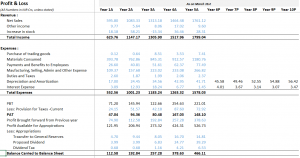Except for the depreciation and interest expense, none of the other line items in the P&L statement are projected. We projected depreciation from the asset schedule and the interest expense from the debt schedule. We will now project the rest of the P&L, which is an easy task.

Starting with revenues, we look at our assumptions for net sales. Recollect, we calculated the year-on-year growth rate of net sales and then projected the average growth rate.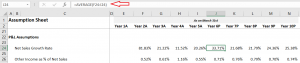We know the net sales for the 5th year and the net sales growth for the 6th year (the projected year); we have to do the math to get the actual value. The math is quite straightforward –

The net sales growth rate for the 6th year = 33.71%

Net sales for 5th year = Rs.1761.12 Crs

Net sales for 6th year = 1761.12*(1+33.71%)

= 2354.71 Crs.

On excel, I’ve calculated using the same approach for all future years –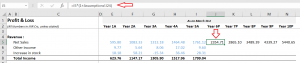One thing that I always make a point to check is the cell linkages. I liked cell J in the P&L sheet and cell J in the assumption sheet. The association is correct, and I need not worry about inadvertent linkage errors.

Once the net sales numbers are in place, we can proceed with other projections since most projections are based on net sales. I’ll demonstrate one of the line items and assume you can do the rest 😊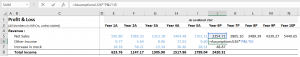We need to multiply the percentage in the assumption sheet with the net sales and get the value. You can see from the screenshot above that I’ve done this for other income and  ‘increase in stock.’ Here is how the fully projected Revenue numbers look –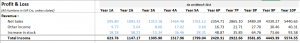We can do the same thing for the expense as well –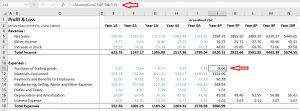Next is the calculation of Profit before tax (PBT), which is essentially the difference between the total income and the total expenses. After calculating the Profit before tax, we need to calculate the tax amount. The tax amount calculation is a very tricky job, and one would need the auditor’s help to arrive at the exact value. Since we must continue the model, we will depend on the averages.

To calculate the average, we have to calculate the tax paid with respect to the PBT in percentage terms. For example, in Y1, the tax paid is 24.15Cr against the PBT of 71.2Cr. In percentage terms,

= 24.15/71.2

= 34%

I can now do the same math across Y1 through Y5 and get the yearly percentages. Once the percentage is in place, I can find out the average across the last five years and treat that as the tax percentage for year 6. You can do this math in one shot in excel –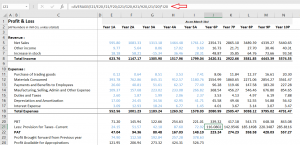Please get comfortable with this technique; we will be using it again shortly. Anyway, we now have the PBT and the provision for current year taxes, PAT of the company is PBT-Taxes, which I’ve calculated.

We are now the last leg of P&L projections. I want you to take a look at this section of P&L  –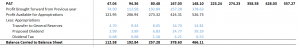The previous year’s Profit is the last year’s closing balance, i.e., ‘balance carried to balance sheet.’ Yes, we apply the base rule again. We now add up the PAT and the Profit available for appropriation to get the total corpus available for allocation.

The transfer to the general reserves is based on the PAT. Here is a tricky part, we have to calculate the appropriation to general reserves, which from P&L goes back to the Reserves schedule. The dividend and dividend tax, too, are calculated. All these calculations are made exactly like how we calculated the tax provision.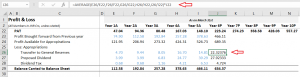Now the closing balance of Rs.634.37Cr for Year 6 is the opening balance for Year 7 and so on. Here is the complete projected P&L for your reference.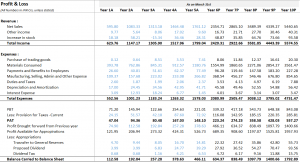We have an old task to complete before moving to the balance sheet projections.

## 12.3 – Reserves Schedule

We were stuck with the reserves schedule because we had no new yearly additions to the general reserves. We can now pull that data from the P&L and complete the reserves schedule.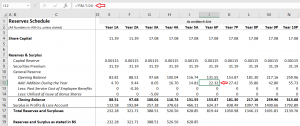Of course, we will pull the reserves schedule data back to the balance sheet. I hope you appreciate how the model is integrated with numbers moving from one sheet to another. The model will only get tighter from here, and even after this many years, I get excited looking at these financial models slowly taking shape 😊

Over to the balance sheet.

## 12.4 –  Balance sheet projections

The balance sheet projection is very similar to the P&L projection. Like the net sales in P&L, the gross block is the alpha line item in the balance sheet. Most of the balance sheet assumptions are based on gross block. I will skip through the few line items in the balance sheet that I think is straightforward and post the snapshot for your reference –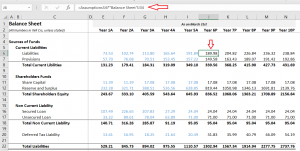Here, current liabilities and current provisions are calculated as a percentage of the gross block. Shareholders’ funds and loans are calculated separately in their respective schedules. Deferred tax liability is calculated as a depreciation percentage (from asset schedule).

Moving ahead, we have the application of funds or the assets side of the balance sheet. The first line item we have to deal with is the inventory. We probably need to spend some time on the inventory.

The inventory value that we see in the balance sheet is the Rupee value of the inventory. We take the inventory data and calculate the ‘Inventory number of days, which is the number of days the company requires to covert the inventory to actual sales. The inventory number of days was calculated in the assumption sheet.

In a sense, the inventory number of days helps us develop an opinion on management’s efficiency, the product’s popularity, market acceptance, etc. For the future years, we take the average of the inventory number of days.

We have the inventory data in Rupee terms in the balance sheet; we have the inventory number of days in the assumption sheet. We also have the inventory number of days for the future years. We now have to convert the inventory number of days for the future years back to the inventory value in the balance sheet. To summarize –

Balance sheet inventory data >> convert to inventory number of day >> project using averages >> convert back inventory number of days back to Rupee value.

The formula to convert inventory number of days back to Rupee value is –

Two*(Inventory number of days * (Material consumed/365))-Previous year inventory.

I will not get into how this formula is derived as that would be a digression; maybe you can look it up online.

I’ve applied the above formula directly on excel (balance sheet), and here is how it looks –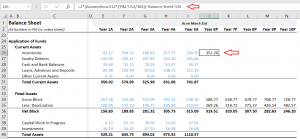The rest of the balance sheet projection is a breeze. I’ll make the projections as per the balance sheet –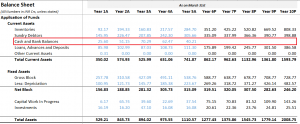Well, congratulations on this mini-milestone. At this point, we have the complete P&L balance sheet projected, except for the cash and bank balance.

We will project the cash and bank balance in the next chapter by building the cash flow statement, which in my opinion, is a significant milestone in our financial model, and there is a reason for that. As you can imagine, the cash and bank balance numbers from the cash flow statement will flow back to the balance sheet. When the cash numbers hit the balance sheet, I’d expect the balance sheet to balance. So, let’s see if that happens in the next chapter 😊

One last thing before we conclude this chapter. We are dealing with so many numbers and projections we are bound to make mistakes. For example, two months after building this model, I may feel that the gross block number for Y6 is 700Cr instead of 588.77Cr; what should I do? Do I have to change the entire model?

No necessary. Since we are building the model in an integrated fashion, we only have to change in one place. The rest of the changes will reflect on their own. So don’t worry too much about the model’s accuracy just yet. We can play around with it as and when we want.

I hope you update your models and bring them up to this level. Do post your queries in the comment section.

### Key takeaways from this chapter

• Most of the P&L and balance sheet projections are straightforward. Take the cues from the assumption sheet.
• Taxes, general reserves, and dividends can be estimated directly in the P&L statement by taking historical ratios and then their average
• The appropriation to general reserves from the P&L statement flows back to the reserves schedule to complete the reserves schedule, which flows back to the balance sheet.
• Inventory is converted to inventory number of days and back to inventory in the balance sheet.
• All line items in the P&L and balance sheet are projected except for the cash and bank balances.
• To project the cash and bank balance, we need the cash flow statement
• The expectation is that the balance sheet gets balanced when the cash and bank balance number flows from the cash flow statement back to the balance sheet
• Since the model is fully integrated, we can change any number in the balance sheet without worrying about its impact on other parts of the financial model.

1.Anivesh says:

Finally sir , eagerly waiting for this ! THANK you sir for uploading this
when you will upload the next chapter ?

•Karthik Rangappa says:

2.Shivansh Agarwal says:

for the deferred tax liability do we have to take the average for the past year and multiply it with the depreciation like we did for general reserves or is it something else

3.Shivansh Agarwal says:

I got it, my bad I didn’t look carefully

•Karthik Rangappa says:

Sure, good luck!

4.Shivansh Agarwal says:

the company which I have taken has one thing called “non controlling interest” in its liabilities side of balance sheet how to deal with it

•Karthik Rangappa says:

Leave it as in if its a one time occurring thing in the balance sheet.

5.Shivansh Agarwal says:

in the inventory formula why have we multiplied it with 2

•Karthik Rangappa says:

Its the formula, dint really want to get into the derivation of it.